The most difficult problems - page 3

1. The sum graphically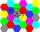Draw a graphically sum of the all sides of 4-gon ABCD.
2. Simple equation 9Solve the following equation: -8y+5=-9y+9
3. Fraction + eqSolve following simple equation with fractions: -5/6(8+5b) = 75 + 5/3b
4. Median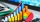The number of missed hours was recorded in 11 pupils: 5,12,6,8,10,7,5,110,2,5,6. Determine the median.There are 15 boys and 12 girls at the graduation party. Determine how many four couples can be selected.
6. Steel tubeThe steel tube has an inner diameter of 4 cm and an outer diameter of 4.8 cm. The density of the steel is 7800 kg/m3. Calculate its length if it weighs 15 kg.
7. Genetic disease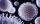One genetic disease was tested positive in both parents of one family. It has been known that any child in this family has a 25% risk of inheriting the disease. A family has 3 children. What is the probability of this family having one child who inherited.
8. Prices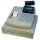The price of the product was increased by 35%. How many percents of the new price we have to make it cheaper so that its price is equal to the original price?
9. GP - three membersThe second and third of a geometric progression are 24 and 12(c+1) respectively, given that the sum of the first three terms of progression is 76 determine value of c
10. Two math problems1) The sum of twice a number and -6 is nine more than the opposite of that number. Find the number. 2) A collection of 27 coins, all nickels, and dimes, is worth \$2.10. How many of each coin are there? The dime, in United States usage, is a ten-cent coin
11. Three members GPThe sum of three numbers in GP (geometric progression) is 21 and the sum of their squares is 189. Find the numbers.
12. An investmentHow long will it take for an investment to double at a simple interest rate of 5.10% p. A. ? Express the answer in years and months, rounded up to the next month.
13. Square ABCDConstruct a square ABCD with cente S [3,2] and the side a = 4 cm. Point A lies on the x-axis. Construct square image in the displacement given by oriented segment SS'; S` [-1 - 4].
14. Camel and water84% of the camel's weight is water. After drinking, its weight increased to 832 kg and water accounted for 85% of its weight. How much did it weigh before drinking?
15. Base of prismThe base of the perpendicular prism is a rectangular triangle whose legs length are at a 3: 4 ratio. The height of the prism is 2cm smaller than the larger base leg. Determine the volume of the prism if its surface is 468 cm2.
16. The second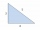The second angle of a triangle is the same size as the first angle. The third angle is 12 degrees larger than the first angle. How large are the angles?
17. Set of coordinatesConsider the following ordered pairs that represent a relation. {(–4, –7), (0, 6), (5, –3), (5, 2)} What can be concluded of the domain and range for this relation?
18. Pine's forest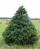There were so many pines in the forest that if they were sequentially numbered 1, 2, 3,. .. , would use three times more digits than the pine trees alone. How many pine trees were there in the forest?
19. Tree trunk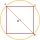What is the smallest diameter of a tree trunk that we can cut a square-section square with a side length of 20 cm?
20. RPMAn electric motor makes 3,000 revolutions per minutes. How many degrees does it rotate in one second?

Do you have an interesting mathematical word problem that you can't solve it? Enter it, and we can try to solve it.

To this e-mail address, we will reply solution; solved examples are also published here. Please enter e-mail correctly and check whether you don't have a full mailbox.